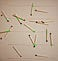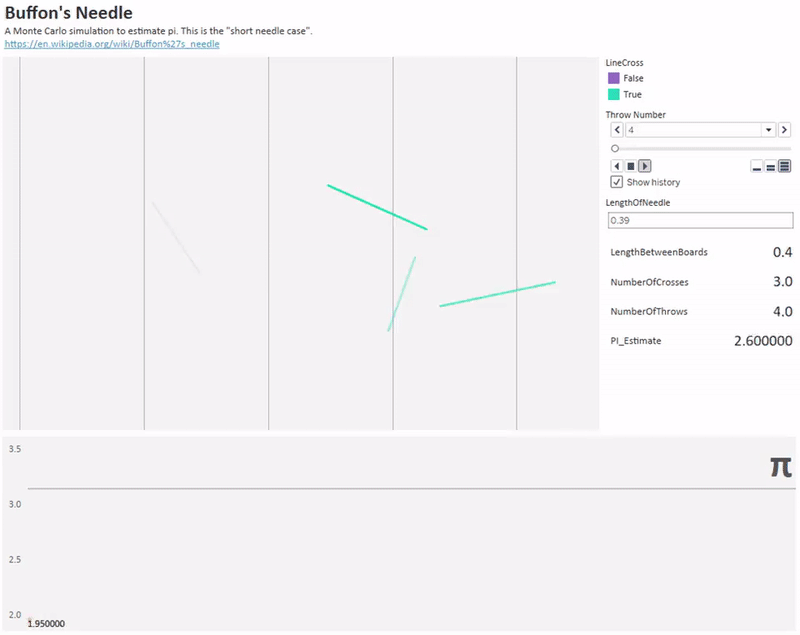Estimate Pi by Dropping Toothpicks Unfortunately most of the students only know pi as related to the circumference of a circle; however, pi is everywhere in nature and in the world of mathematics. One of the best examples to prove this statement is Buffon’s needle experiment, which is about statistics.Above: An experiment to find π, via Wikipedia It is a super simple but a really cool math project to do and it’s about probability. Your students can find pi by only throwing dropping toothpicks on aboard. First, you measure the length of the toothpicks and draw lines and make the distance between lines exactly the same as the length of the toothpicks. Then you drop your toothpicks and count how many of them crossing the lines and how many of them not crossing the lines. Then pi will be equal to 2 * (the number of times toothpick you drop / the number of toothpicks crossing the line times). Researchers at the University of Illinois made a simulation to virtually drop needles and estimate pi.Above: Simulation of throwing 1000 needles to estimate pi, via Reddit • Project Type: Probability & Data Science • Supplies: A board, toothpicks, a marker